# Introduction to Probability

Go back to  'Probability'

# Probability

We talk a lot about probability in our every day life, knowing or unknowingly. Each of us has some intuitive notion of this concept. For example, ponder over these statements:

“ There is a good chance that it will rain today ”

“ India is almost sure to win this T20 match against Pakistan ”

“ The stock market is likely to fall again soon ”

“ Issac Newton is probably the most influential human to have ever existed ”

We make innumerous statements of these sort everyday. There is an underlying “chance” element in each such statement. Have you ever paused and wondered why this chance element arises, and how our minds are able to quantify this chance element intuitively, without any mathematical knowledge whatsoever? If you haven’t, this chapter gives you a chance to do so!

# Motivation  And  Introduction

The aim of this chapter is to make you understand how probabilities are calculated. We will, of course, use our intuition in this task as much as possible, but you’ll see in some cases that our intuition in matters of probability can be off the mark!

Let us see some examples from everyday life that’ll give you an idea of the mathematical significance of probability and the necessity of calculating exact probabilities. We will, for the time being, not worry too much about the issue of defining probability rigorously in a mathematical sense; we’ll postpone that matter to some point later in the chapter; for now, we’ll keep the discussion intuitive.

Scenario - 1:

You are playing a game with your friend, which involves rolling a single die once. The rules of the game are that you’ll win if an even number shows up while your friend will win if an odd number shows up.

It should be immediately apparent to you that both you and your friend are equally likely to win this game. Why? Because you win in three possible cases, i.e, if one of the numbers 2, 4 or 6 shows up, while your friend wins if one of 1, 3 or 5 shows up. Since for a (fair) die, each of the six faces is equally likely to show up, we can safely state that since both of you have 3 favorable cases to your credit respectively, both of you are equally likely to be the winner.

Can we somehow quantify this discussion? That is, can we somehow assign numerical values to the various chances involved? It turns out that we can, and in a way that is very intuitively appealing, as follows:

Technically, we term any incident, an event (we will define events more precisely later; for the time being, just think of an event as an incident). Now, if an event E is sure to occur, we say that the probability of the occurrence of E is 1, and we write this as

$P\left( E \right)=1$

On the other hand, if an event F is sure not to occur, we say that the probability of the occurrence of F is 0, and we write this as

$P\left( F \right)=0$

For any event G that is likely to happen, we should then have

$0\le P\left( G \right)\le 1$

which means that the probability of G occurring must lie between 0 and 1; it can be 1 at the most which implies that G is sure to occur; it can be 0 at the least which implies that G is sure not to occur.

Here are some simple examples:

Event E  :  The sun will rise in the east tomorrow

Event F  :  The sun will rise in the west tomorrow

Event :  You’ll obtain a “Heads” upon tossing a fair coin

Thus, we must have,

P (E) = 1  ;  E is sure to occur

P (F) = 0  ;  F cannot occur under any circumstance.

0 < P(G) < 1  ;  You can’t say for sure that you will definitely obtain a “Heads”. Thus, P(G) cannot be 1. Similarly, P (G) cannot be 0 either since you can’t say for sure that you will definitely not obtain a “Heads”.

P (G) therefore must lie somewhere between 0 and 1; where exactly we’ll soon understand!

Coming back to the die-game you and your friend were playing, let us assign a numerical value to the chance of any of the six faces showing up.

This should be easy! There are six faces, and it is easy to observe that each of these six faces is equally likely to show up, which means that there is no reason why we should believe one face to be more likely to turn up than any other.

Thus, if we let $$P(i)$$ denote the probability of the $${{i}^{th}}$$ face turning up, we must have,

$P\left( 1 \right)=P\left( 2 \right)=P\left( 3 \right)=P\left( 4 \right)=P\left( 5 \right)=P\left( 6 \right)\cdot\cdot\cdot\cdot\cdot\cdot\cdot\cdot\cdot\cdot\cdot\cdot\cdot\cdot(1)$

What we’ve stated till now is just that each of the six faces is equi-probable; we still haven’t obtained the numerical values to these probabilities.

To do so, observe that one of the six faces must show up: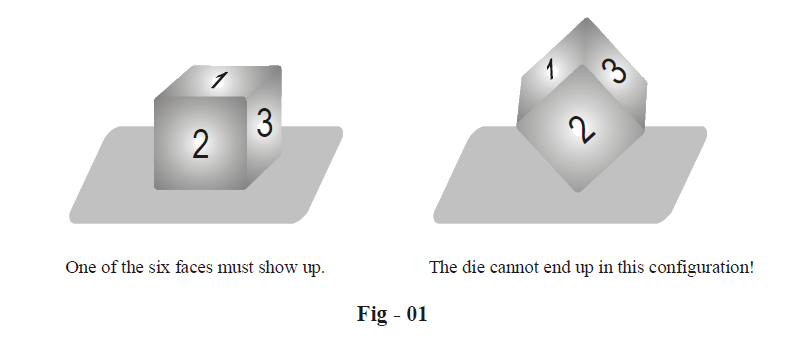Thus, we can intuitively assert that the probabilities of the six faces showing up must sum to 1, because one face must show up. This means that

$P\left( 1 \right)+P\left( 2 \right)+P\left( 3 \right)+P\left( 4 \right)+P\left( 5 \right)+P\left( 6 \right)=1\quad\cdot\cdot\cdot\cdot\cdot\cdot\cdot\cdot\cdot\cdot\cdot\cdot(2)$

From (1) and (2), we have

$P\left( 1 \right)=P\left( 2 \right)=P\left( 3 \right)=P\left( 4 \right)=P\left( 5 \right)=P\left( 6 \right)=\frac{1}{6}$

So far, so good! However, we’ve still not given any rigorous justification for (2). For now, you’ll have to accept the validity of (2) on faith.

Now, the game’s rules were that you’ll win if an even number turns up. Thus, if we let E denote the event of you being the winner, we can safely state (according to what we’have been doing till now) that,

\begin{align}& P\left( E \right)=\,\,\,P\left( 2 \right)+P\left( 4 \right)+P\left( 6 \right) \\ &\quad \quad\;\,=\,\,\,\;\frac{1}{6}\,\,\,\,\,\,+\,\,\,\,\,\frac{1}{6}\,\,\,\,\,+\,\,\,\frac{1}{6} \\ & \quad\quad\;\,=\,\,\,\frac{1}{2} \end{align}

Similarly, if we let F denote the event that your friend wins, we should have

\begin{align}& P\left( F \right)=\,\,\,P\left( 1 \right)+P\left( 3 \right)+P\left( 5 \right) \\ &\quad\quad\;\, =\,\,\,\frac{1}{6}\,\,\,\,\,\,\,\,\,+\,\,\,\,\,\frac{1}{6}\,\,\,\,\,+\,\,\,\frac{1}{6} \\ &\quad\quad\;\, =\,\,\,\frac{1}{2} \\ \end{align}

Thus, both you and your friend have a probability \begin{align}\frac{1}{2}\end{align} of winning the game. This should have been otherwise obvious also! Since E and F must be equi-probable, and we also have

$P\left( E \right)+P\left( F \right)=1,$

we must have

$P\left( E \right)=P\left( F \right)=\frac{1}{2}$

Well, the preceeding discussion was too long for too simple an example. But it was necessary to get you tuned to the flavor of this subject.

Scenario - 2: You toss a fair coin thrice. Since there are two outcomes at each toss, namely “Heads” and “Tails” the total number of possible outcomes is

$2\times 2\times 2=8$

Let us list down all the 8 outcomes for clarity’s sake; H represents “Heads ” and T represents “Tails”:

$HHH,\quad HHT,\quad HTH,\quad THH$

$HTT,\quad THT,\quad TTH,\quad TTT$

Since the coin is fair, we have sufficient reason to believe that each of these 8 outcomes is equally likely, i.e. equi-probable. Also, since these are the only outcomes possible and one of them must occur, we can say that the probability of any outcome is \begin{align}\frac{1}{8}\end{align} . For example,

$P\left( HHT \right)=P\left( TTH \right)=\frac{1}{8}$

Now, let us pose the following question: in the sequence of these three throws, what is the probability of H occuring before T?

This is easy; we count all cases satisfying the stated constraint:

$HHH,\quad HHT,\quad HTH,\quad HTT$

Since there are 4 “favorable” cases to our constraint out of the total 8 cases possible, it should be correct to say that the possibility of H occuring before T should be \begin{align}\frac{4}{8}=\frac{1}{2}\end{align}  . Similarly, the possibility of T occuring before H should also be  \begin{align}\frac{4}{8}=\frac{1}{2}\end{align}.

Now let us try to answer the same question from a slightly different perspective. Let us define the events E and F as follows for this sequence of three tosses:

E : H occurs before T

F : T occurs before H

The task is to find P(E) and P(F), which we earlier did by explicitly counting the favorable cases. Here we’ll try to calculate P(E) and P(F) differently. Observe that one of the events E or F must occur; there is no other possibility. If we denote the outcomes of our “experiment” (involving the sequence of three tosses), by a rectangle, we can divide the rectangle into exactly two halves, one representing the event E and one the event F.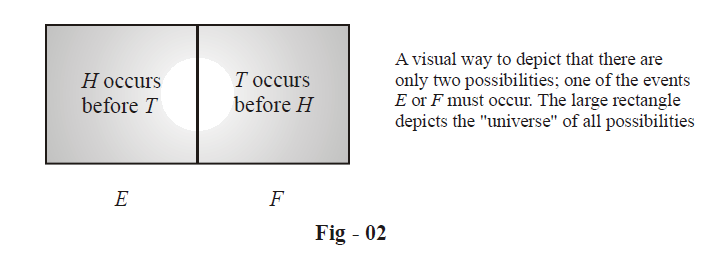Now, it should be obvious that

$P\left( E \right)+P\left( F \right)=1\quad....... (3)$

Also, since the coin is fair, E and F must be equi-probable. This is because H and T are equi-probable, i.e, equivalent outcomes in terms of probability. Thus, H occuring before T must be as likely as T occuring before H. Hence, we must have

$P\left( E \right)=P\left( F \right)\quad...... (4)$

From (3) and (4), we must have

$P\left( E \right)=P\left( F \right)=\frac{1}{2}$

Let us now pose a further question for this sequence of three tosses. Let the events X and Y be defined as follows:

X : There are more H than T

Y : There are more T than H

Our task is to calculate P(X) and P(Y). These should be immediately apparent. Since there are an odd number of tosses, one of the events X or Y must occur. Also, X and Y have to be equi-probable.

Thus,

\begin{align}&\qquad\quad P\left( X \right)+P\left( Y \right)=1\,\;\;\;and\,\;\;P\left( X \right)=P\left( Y \right) \\ & \Rightarrow \quad P\left( X \right)=P\left( Y \right)=\frac{1}{2} \\ \end{align}

Your are urged to arrive at the same result by the explicit counting of out comes.

Scenario - 3: Suppose that you have with you a standard deck of 52 cards, and you shuffle it really well. You now draw a card at random from this deck. It is obvious that for a well-shuffled deck, you are equally likely to draw any of the 52 cards. For example, if E is the event that the card drawn is the king of Hearts while F is the event that the card drawn is the seven of Diamonds, we have

$P\left( E \right)=P\left( F \right)$

Since there are 52 cards and each is equally likely to turn up, and also, one of the 52 cards must turn up, the numerical value of the probability of any card being drawn is therefore \begin{align}\frac{1}{52}\end{align} . For example,

$P\left( E \right)=P\left( F \right)=\frac{1}{52}$

Now, let G be the event that a spades is drawn. Since G can occur in 13 ways (there are 13 cards in any suit), or in other words, the number of cases favorable to G is 13 out of the total 52 possibilities, we must have

$P\left( G \right)=\frac{13}{52}=\frac{1}{4}$

This value of  $$P\left( G \right)$$ should have been obvious directly, since there are only 4 suits in the deck, and any suit is equally likely to turn up.

Going further, let H be the event that a black card is drawn. We have (# represents ‘Number of’),

\begin{align}&\quad\qquad\#\text{Blackcards}\,\,\,\mathbf{=}\,\,\,\,\,\#\,\,\text{Spades}\;\;\;\text{+}\,\,\,\,\,\text{ }\!\!\#\!\!\text{ }\,\,\text{Clubs} \\\\ & \Rightarrow \quad \frac{\#\text{Black cards}}{\text{ }\!\!\#\!\!\text{ }\,\text{All cards}}=\,\,\,\frac{\#\,\,\text{Spades}}{\text{ }\!\!\#\!\!\text{ }\,\text{All cards}}+\,\,\,\frac{\#\,\,\,\text{Clubs}}{\,\text{ }\!\!\#\!\!\text{ }\,\text{All cards}} \\ & \Rightarrow \qquad \frac{26}{52}\qquad\qquad=\qquad \frac{13}{52}\qquad+\qquad\frac{13}{52} \\ \end{align}

We see that  \begin{align}P\left( H \right)=\frac{26}{52}=\frac{1}{2}\end{align} , since there are 26 cases favorable to H. Again, the value of P(H) should have been obvious directly, since there are only 2 colors in the deck (red, black), and each color is equally likely to turn up.

From this discussion, you should by now have understood the gist of the concept of probability. We can, taking cue from the preceeding examples, define the probability of an event as the number of outcomes favorable to that event, divided by the total number of possible outcomes (assuming each outcome to be equally likely).

Extending this further, the general idea is that if we have two events E and F with no outcomes in common, the first consisting of x outcomes and the second of y outcomes, then the event G which consists of all the outcomes in E and F consists of x + y outcomes, i.e,

$\#G=\#E+\#F\;(\text{if E and F have no outcomes in common})$

An example of this is what we just saw:

# Black cards = # Spades + # Clubs

Thus, if the total number of outcomes is Z,

we have,

\begin{align} & P\left( G \right)=\frac{\#G}{Z} \\\\ &\quad\quad\;\; =\frac{\#E+\#F}{Z} \\\\ & \quad\quad\;\;=\frac{x}{z}+\frac{y}{z} \\\\ & \quad\quad\;\;=P\left( E \right)\text{ }+P\left( F \right) \\ \end{align}

That the probabilities add when we consider the event G consisting of all outcomes in E and F is justified only if E and F have no outcomes in common, as we’ve been seeing till now.

What about the probability of an event consisting of all outcomes in two events E and F which may have some outcomes in common? For example, let E and F be events defined as follows:

E : The card drawn is even

F : The card drawn is black

The number of outcomes in E is 24 (why ? ). The number of outcomes in F is 26. However, if we now consider the event G which consists of all the outcomes in E and F, we find that the relation

$\#G=\#E+\#F$

is not satisfied. Why? Because the events E and F have some outcomes in common. Let us understand this visually.

We let the large rectangle below represent all the 52 possible outcomes. The events E and F are then subsets of this large rectangle as shown.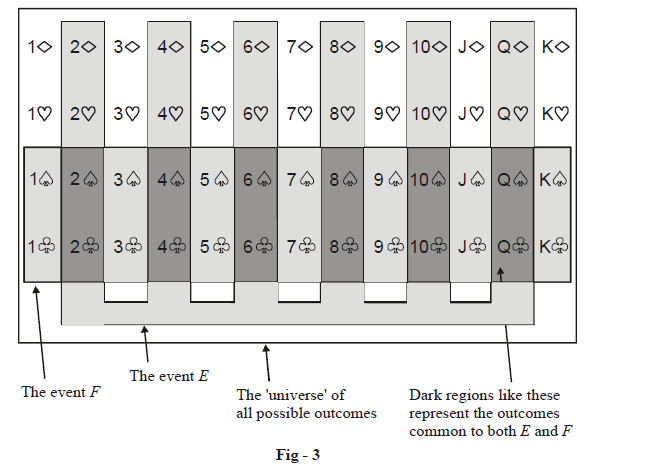Note that E and F have some elements in common, which is technically stated by saying that E and F are not mutually exclusive. (Thus, mutually exclusive events have no outcomes in common).

As stated earlier, the event G is defined to consist of all the outcomes in E and F, which (in easier language!) means that G is the event that the card drawn is either even or black (or both). How many elements are there in G?

Note that when we write

$\#G=\#E+\#F\,\,\left( ✗\text{ }not\text{ }correct\text{ } \right)$

the outcomes common to E and F (represented by the dark regions in Figure - 3) are counted twice on the right side. The actual number of outcomes in G will thus be obtained by subtracting from the RHS the number of these common outcomes, and thus we can write

$\#G\text{ }=\#E\text{ }+\text{ }\#\text{ }F-\text{ }\#\text{ }EF\left( \checkmark \text{ }not\text{ }correct\text{ } \right)$

where #EF represents the number of common outcomes. In this case, EF would represent the event that the card drawn is both even and black. There are obviously 12 such cards, as is evident from Figure - 3, and thus,

\begin{align}& \#G\,\,=\,\,\,\,24\,\,\,\,+\,\,\,\,\,26\,\,\,\,\,\,\,\,\,\,12 \\ &\,\,\,\,\,\,\,\,\,\,\;\;\;\#E\,\,\,\;\;+\,\,\,\,\#F\,\,\,\,\,\,\,\#(EF) \\ &\quad\quad =38 \\ \end{align}

You are urged to verify this result by explicitly counting the number of cards lying within the (total) shaded regions in Figure - 3.

In passing, it should be mentioned that the event G which has been defined as the event consisting of all the outcomes in E and F is technically termed as the union of the events E and F, and this fact is symbolically written as

$\boxed{G\,\, = \,\,E\, \cup \,F} \qquad\qquad \text{Union of events}$

You can think of the union of two events to be the event which can be said to occur when either of the two given events (or both) occurs.

Analogously, the event H defined to consist of all outcomes common to E and F is technically termed as the intersection of the events E and F, and this fact is symbolically written as

$\boxed{H\,\, = \,\,E\,\, \cap \,\,F}\qquad\qquad \text{Intersection of events}$

You can think of the intersection of two events to be the event which can be said to occur when both the two given events occur simultaneously. Finally, as we’ve seen above, we always have

$\boxed{\# \left( {E\,\, \cup \,\,F} \right)\,\, = \,\,\# E\,\, + \,\,\# F\,\,-\,\,\# \,\left( {E\,\, \cap \,\,F} \right)}\qquad\qquad........ (5)$

If E and F have no outcomes in common, that is, if E and F are mutually exclusive:

$\left( {\# \,\left( {E\,\, \cap \,\,F} \right)\,\, = \,\,0} \right),$

this relation reduces to

$\boxed{\# \left( {E\,\, \cap \,\,F} \right)\,\, = \,\,\# E\,\, + \,\,\# F}\qquad\qquad....... (6)$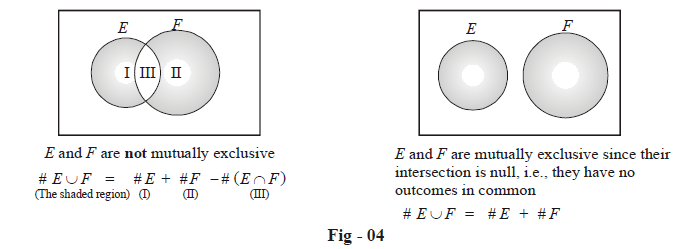The relations (5) and (6), when written in terms of probability (by dividing both sides with the total number of outcomes), become,

$\boxed{P\left( {E\, \cup \,F} \right)\,\, = \,\,P\left( E \right)\,\, + \,\,P\left( F \right)\,\,-\,\,P\left( {E\,\, \cap \,\,F} \right)} \qquad\qquad \text{General relation }... (7)$

$\qquad\qquad\qquad\qquad\boxed{P\left( {E\, \cup \,F} \right)\,\, = \,\,P\left( E \right)\,\, + \,\,P\left( F \right)} \quad\quad \text{The particular case of E and F being mutually exclusive }... (8)$

If the concept of mutually exclusive events is now clear to you, the significance of the relation (2) on Page - 3 immediately becomes apparent, where we simply summed the six probabilities and wrote the sum as 1. All the six outcomes are mutually exclusive, and they exhaust all possibilities, so (2) is justified.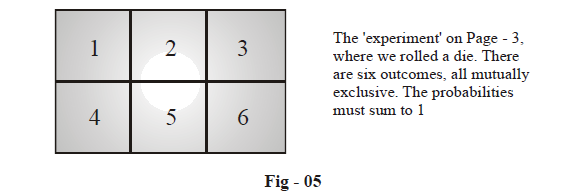Now is a good point to learn some more terminology. In Figure - 5 above, each of the six possible outcomes is the most elementary outcome possible. For example, if E is the event of a 4 showing up, the event E consists of only one outcome, the number 4. E would therefore be called an elementary event. Now consider the event F of an odd number showing up. F consists of three outcomes, namely 1, 3 and 5. F would therefore be termed a compound event.

As a further example, in the drawing of a card at random from a standard deck of 52 cards, let G and H be events defined as follows:

G : The card drawn is the king of Hearts

H : The card drawn is a Hearts

G is an elementary event since G consists of only one possible outcome, the king of Hearts (stated differently, # G = 1). However, H is a compound event since H consists of 13 possible outcomes, namely the 13 cards of Hearts. In other words, # H = 13.

Scenario - 4: In the experiment of drawing one card at random from a standard well-shuffled deck of 52 cards, consider the event E defined as:

E : The card drawn is a King

Obviously, we have

\begin{align}& P\left( E \right)=\frac{\#\text{Kings}}{\#\text{All}\,\text{cards}} \\ &\quad\quad\;\,=\frac{4}{52} \\ &\quad\quad\;\, =\frac{1}{13} \\ \end{align}

However, if you are now asked to find the probability of event E occurring given that the card drawn has a number greater than 10, what would you say?

To answer correctly, you must understand that now the situation is entirely different from earlier. Now we already know that the card drawn has a number greater than 10, which means that the number of possibilities for the card has reduced; earlier, there were 52 possibilities, but now the number of possible cards are the 4 Jacks, the 4 Queens and the 4 Kings, which means that now there are only 12 possibilities for the card. Of these, the favorable possibilities are 4 ; thus,

\begin{align}& P\left\{ \begin{gathered}\text{Event }E\text{ given that the card drawn } \\ \text{has a number greater that 10} \\ \end{gathered} \right\}=\frac{\#\,\,\text{Kings}}{\text{ }\!\!\#\!\!\text{ Reduced possibilities}} \\ &\qquad\qquad\qquad\qquad\qquad\qquad\qquad\qquad\qquad =\frac{4}{12} \\ & \qquad\qquad\qquad\qquad\qquad\qquad\qquad\qquad\qquad=\frac{1}{3} \\ \end{align}

Let us denote by F the event that the card drawn has a number greater than 10. The probability just calculated above is then written in standard notation as

$P\left( E/F \right)$

$P\left( E\,\,\text{given}\,\,F \right)$

i.e.

“the probability of event E occurring given that F has occurred”.

Let us consider another example. In the random experiment of throwing two dice, let events G and H be defined as

G : The sum of the two numbers on top is 6

H : One of the numbers on top is 4

First, let us find the probability of G occurring. This is simply

\begin{align}& P\left( G \right)=\frac{\#\text{favorable}\,\,\text{possibilities}}{\#\,\text{total}\,\,\text{possibilites}} \\ &\quad\quad\; =\frac{\left( 1,5 \right)\,\,\left( 2,4 \right)\,\,\left( 3,3 \right)\,\,\left( 4,2 \right)\,\,\left( 5,1 \right)}{6\times 6} \\ &\quad\quad\; =\frac{5}{36} \\ \end{align}

Now, suppose we are given that H has already occurred, meaning we now know that one of the numbers on top is 4. What is the probability of G now when we already possess this information?

The number of total possibilities now are 11; we list them explicitly:

$\left\{ \begin{matrix}\left( 4,1 \right) & \left( 4,2 \right) & \left( 4,3 \right) & \left( 4,4 \right) & \left( 4,5 \right) & \left( 4,6 \right) \\\left( 1,4 \right) & \left( 2,4 \right) & \left( 3,4 \right) & {} & \left( 5,4 \right) & \left( 6,4 \right) \\\end{matrix} \right\}$

Out of these, the favorable possibilities are only 2, namely: (4, 2) and (2, 4). Thus

\begin{align}& P\left( G/H \right)=\frac{\#\text{favorable}\,\,\text{possibilities}}{\#\text{reduced possibilities}} \\ &\qquad\qquad =\frac{2}{11} \\ \end{align}

In both the preceeding examples, what happens is that with the possession of some information, the number of possibilities reduce, i.e., the sample space reduces. In the first example, with the information that F has occurred, the number of possibilities reduces from 52 to 12. We then looked for favorable cases only within this reduced sample space of 12 outcomes. Similarly, in the second example, when we possess the information that H has occurred, the number of possibilities reduces from 36 to 11; it is in this reduced sample space of outcomes that we then looked for the favorable possibilities.

In passing we should mention that probabilities of the form P(A/B) are called conditional probabilities. P(A/B) is said to be the conditional probability of A given that B has occurred.

Let us understand all this somewhat more deeply. In particular, let us ponder over the following question: Let E and F be two events. Let the probability of E occurring be P(E). Now, if we come to know that F has occurred, will the probability of E occurring always increase, like it did in the last two examples, or can it decrease too? Can it remain the same?

It turns out that all the three are possible, which should even be obvious to an alert reader.

Let us consider the case where the information that F has occurred decreases the probability of E occurring. Let E and F be, in the card drawing experiment of the first example

E : The card drawn has a number which is a multiple of four

F : The card drawn has a number greater than eight

The original probability of E occurring is

\begin{align}& P\left( E \right)=\frac{\#\text{favorable}\,\,\text{possibilities}}{\#\,\text{total}\,\,\text{possibilites}} \\\\ &\quad\quad\; =\frac{\left\{ 4,8,12 \right\}\text{per suit}\,\,\times \,\,\text{4}\,\,\text{suits}}{52} \\\\ &\quad\quad\; =\frac{12}{52}\,\,=\,\,\frac{3}{13} \\ \end{align}

But when F has already occurred, the probability of E occurring is

\begin{align}& P\left( E/F \right)=\frac{\#\text{favorable}\,\,\text{possibilities}}{\#\,\,\text{reduced}\,\,\text{possibilites}} \\\\ &\qquad\quad \;\;=\frac{4\,\,\text{Queens}}{\left\{ 9,10,J,Q,K \right\}\text{ per suit }\times \,\,4\,\text{suits}} \\\\ &\qquad\quad \;\; =\frac{4}{\text{20}}=\frac{1}{5} \\ \end{align}

which is lesser than the original probability of E occurring. This should be intuitively obvious: In the first case, there are 3 multiples of four per suit of 13 cards. In the second, when we are told that the number of the card is greater than eight, the multiple of four possible is only 1 per suit which is the queen. Thus the favorable possibilities decrease to one-third. The total possibilities also decrease i.e, from 13 to 5 per suit, but it can be easily appreciated that the percentage reduction in favorable possibilities is greater than the percentage reduction in total possibilities.

We now come to the third very important question: for two events E and F, can P(E / F) be the same as P(E)? You must appreciate that this is equivalent to saying that the probability of E occurring is not affected by the occurrence or non-occurrence of F.

As we said earlier, this is possible, and such events are called independent events:

If P(E / F) = P(E)

$$\Rightarrow$$   E and F are independent events

Let us see an example. In the card-drawing experiment, let events E and F be defined as

E : The card has a number greater than eight

F : The card is black

The alert reader will immediately realize that P(E / F) is the same as P(E). Why? Because, the knowledge that the card is black does not change the number of cards per suit that are greater than eight. Stated explicitly,

\begin{align}& \quad\qquad P(E)=\frac{\{9,\ 10,\ J,\ Q,\ K\}\ \text{per}\ \text{suit}\ \times \ 4\ \text{suits}}{52} \\ &\quad\qquad \quad\quad\;\,=\frac{20}{52}=\frac{5}{13} \\ & and\quad P(E/F)=\frac{\{9,\ 10,\ J,\ Q,\ K\}\ \text{per}\ \text{suit}\ \times 2\ \text{suits}}{26}\,\,\left\{ \begin{gathered} \text{because}\,\text{there}\,\text{are } \\ \text{only}\,\text{two black}\,\text{suits} \\ \end{gathered} \right\} \\ &\qquad\qquad\qquad =\frac{5}{13} \\ \end{align}

There is one important point the reader must notice and appreciate:

\begin{align} & \text{If }P\left( E/F \right)\text{ }=P\left( E \right)\text{ }\to \text{this means that E and F are independent events} \\ & \ \ \; \; \; \; \; \; \; \; \; \;\; \; \; \; \; \; \;\; \; \; \; \,\,\,\,\,\,\,\,\,\,\,\,\,\to \text{this should also mean that }P\left( F/E \right)\text{ should be the same as }P\left( F \right) \\ \end{align}

Let us verify this in the example above. We have,

\begin{align} & \qquad\qquad\qquad P(F)=\frac{\#\ \text{black}\ \text{cards}}{\#\ \text{total}\ \text{cards}} \\\\ & \qquad\qquad\qquad\qquad\;=\frac{26}{52}=\frac{1}{2} \\\\ & \text{and},\qquad\;\,\,P(F/E)=\frac{\#\ \text{black}\ \text{cards}\ \text{greater}\ \text{than}\ \text{eight}}{\#\ \text{total}\ \text{cards}\ \text{greater}\ \text{than}\ \text{eight}} \\\\ &\qquad\qquad\qquad \qquad \,\,=\frac{\{9,\ 10,\ J,\ Q,\ K\}\ \text{of}\ \text{Spades}\ \text{and}\ \text{of}\ \text{Clubs}}{\{9,\ 10,\ J,\ Q,\ K\}\ \text{per}\ \text{suit}\ \times \ 4\ \text{suits}} \\\\ &\qquad\qquad\qquad\quad\quad\; =\frac{10}{20} \\\\ &\qquad\qquad \qquad\qquad\;=\frac{1}{2} \\ \end{align}

which confirms the assertion

$\boxed{\begin{gathered}\;{\text{IF}}\;A\;{\text{and}}\;B\;{\text{are}}\;{\text{independent}}\;{\text{events}}\\\;\;\;\;\;\;\;\;\;\; \Rightarrow \,\,\,P(A/B) = P(A)\\ \;\;\;\;\;\;\;\;\,{\text{and}}\;P(B/A) = P(B) \end{gathered} }\qquad\qquad....... (1)$

Note that the favorable cases while calculating P(A / B) are those cases in A that are common to B; the total cases are all cases in B. Thus

\begin{align}&P(A/B) = \frac{{\# \;{\text{favorable}}\;{\text{cases}}}}{{\# \;{\text{total}}\;{\text{cases}}}}\\&\qquad\quad\;\, = \frac{{\# \;(A \cap B)}}{{\# \;(B) }} \quad\quad...(2)\end{align}

If the entire sample space of the experiment consists of N out comes, we can write (2) as

\begin{align}& P(A/B)=\frac{\#\ \left( A\cap B \right)/N}{\#\ \left( B \right)/N} \\ &\qquad\quad \;=\frac{P\left( A\cap B \right)}{P\left( B \right)}\quad\quad.......(3) \\ \end{align}

Similarly,

$P\left( B/A \right)=\frac{P\left( A\cap B \right)}{P\left( A \right)}\quad\quad...(4)$

This means that if A and B are independent, then

\begin{align} &\quad \qquad P\left( {A/B} \right) = P(A) = \frac{{P\left( {A \cap B} \right)}}{{P\left( B \right)}}\,\,\,\left( {From{\text{ }}\left( 1 \right){\text{ }}and{\text{ }}\left( 3 \right)} \right) \\ & \Rightarrow \quad \boxed{\;P\left( {A \cap B} \right) = P(A) \cdot P(B)\;} \end{align}

Thus, the probability of the intersection of two independent events is simply the product of the individual probabilities.

Before moving on, some very important remarks need to be made:

(1) If events A and B are from two different sample spaces, then they are obviously independent. For eg, let us consider the experiment of throwing a coin and a die simultaneously. Any outcome of the coin throw is independent    of any outcome on the die roll.

(2) Do not confuse mutually exclusive events with independent events! In fact,

If A and B are two non-impossible mutually exclusive events, then they are not independent.

Reason: This should be obvious. If A and B are ME events, then the occurrence of one rules out, i.e, affects, the occurrence of the other which means that they are not independent. Mathematically speaking,

We have

$P(A) \ne 0,\;P(B) \ne 0,\;{\text{but}}\;P(A \cap B) = 0$

$\text{because} \;\;A \cap B = \phi\;.$

$\text{Thus}, P(A \cap B) \ne P(A) \cdot P(B)$

If A and B are two non-impossible independent events, they they are not mutually exclusive.

Reason: This is again very simple to understand. If A and B are independent events then obviously the occurrence of one does not rule out the occurrence of the other, which means that they are nor ME. Mathematically,

\begin{align} &\qquad\qquad\quad P(A)\ne 0,\ P(B)\ne 0 \\ & \text{Thus},\qquad P(A\cap B)=\ P(A)\cdot P(B)\ne 0 \\ & \text{which means}\;A\cap B\ \text{is}\;\text{not}\ \ \phi \\ \end{align}

Try to understand these two assertions with actual examples.

We now consider one final example before going on to the next section.

Scenario – 5: You are sitting in a class of 32 students, and your eccentric Math Professor is teaching you probability. Suddenly, he pops this statement at the whole class:

“You know what! There is a more than 75% chance that at least two students in this class share the same birthday!”

What would your reaction be? Would you term his assertion crazy? After all, the class has only 32 students while a year has 365 days. That the chances of two students sharing the same birthday in such a small class could be greater than 75% (which is pretty high!), will definitely strike one as strange.

However, it turns out that your professor is correct. Let us try to understand how. Later on, we’ll revisit this same problem with more rigor.

Considering that there is no time of the year when there is a sudden ‘baby boom’ where the rate of babies being born jumps abruptly, it is fair enough to assume that any random person you choose is equally likely to born on any day of the year. Thus, for example, each of the 32 students in your class is equally likely to be born on any day of the year.

Let us first find the total number of ways in which these 32 students could have been born during the year, or more precisely, the number of ways in which these 32 students can be assigned birth-dates. This number is simply

\begin{align}&N=\underbrace{365\times 365\times 365\times ........................\times 365}_{32\,\,\text{times}}\\&\quad =\;{{365}^{32}}\end{align}

by the fundamental principle of counting since each student can be assigned a birth-date in 365 ways. We now count the number of ways in which at least two students could share a birthday. However, you’ll appreciate that it will be easier to analyse the complementary case, namely finding the number of ways of assigning birth-dates to the students so that no two students share the same birthday. This number is simply $$^{365}{{P}_{32}},$$ since from amongst 365 birth-dates, we need to make unique assignments to the 32 students. Thus, the probability of no two students sharing the same birthday will be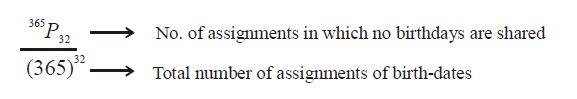which equals 246.

Thus, the probability of the complementary event, i.e. of at least two students sharing a birthday, will be

1 – .246 = .753 or 75.3%. Surprising!

Let us summarize what concepts we’ve encountered upto now in one form or the other. Note: this summary is exceptionally important. Make sure that you have a good idea about what all’s involved.

Concept/Terminology        :        Example / Explanation

(i) Outcome     :   The result of a random experiment. For example, in the tossing of a coin, obtaining “Heads” is a possible outcome

(ii) Equally likely     :  Two outcomes A and B of an experiment can be said to be equally likely when there is no evident reason to favor A over B or viceversa. To make the idea more concrete, you can say that as you repeat the experiment an indefinitely large number of times, the relative occurrence of A and B will be equal. For example, if you toss a fair coin an indefinitely large number of times, the relative occurrence of both Heads and Tails will be \begin{align}\frac{1}{2}\end{align} . Similarly, if a die is rolled an indefinitely large number of times, each of the six faces will have a relative occurrence of  \begin{align}\frac{1}{6}\end{align}

(iii)  Event   :   An event is a set of outcomes. Thus, an event can be viewed as a subset of the universe of all outcomes of the experiment, which is termed as the sample space of the experiment.

For example, in drawing a card from a well-shuffled deck of 52 cards at random, the sample space is of size 52. The event E defined as

E : The card drawn is red

is a set of 26 outcomes.

The event F defined as

F : The card drawn is a king

is a set of 4 outcomes.

The event G defined as

G : The card drawn is the Ace of spades

is a set of only 1 outcome, and is thus an elementary event, whereas E and F are compound events.

(iv) Probability   :   If all outcomes in an experiment are equally likely (like in tossing a fair coin, rolling a fair die, drawing a card at random from a well-shuffled deck), then the probability of occurrence of an event E is simply

$P\left( E \right)\,\,=\,\,\frac{\text{No}\text{.}\,\,\text{of}\,\,\text{outcomes}\,\,\text{favorable}\,\,\text{to}\,\,E}{\text{Total}\,\,\text{No}\text{.}\,\,\text{of}\,\,\text{outcomes}}$

Note that  $$P\left( E \right)\,\,\in \,\,\left[ 0,\,\,1 \right]$$

(Note also that probability defined this way is not applicable to situations like weather prediction, which use advanced probabilistic models).

(v) Events as sets :  Since events are sets of outcomes, set operations can be defined for events. Let E and F be two events associated with a random experiment. In general, we have

$\#\left( E\,\,\cup \,\,F \right)\,\,=\,\,\#E\,\,+\,\,\#F\,\,-\,\,\#\left( E\,\,\cap \,\,F \right)$

In case of mutually exclusive events, this reduces to

$\#\left( E\,\,\cup \,\,F \right)\,\,=\,\,\#E\,\,+\,\,\#F\,$

(vi) Conditional Probability :  If E and F are two events, then the probability of E occurring given that F has already occurred is termed the conditional probability of E given F, and is written as P(E / F). We have,

$P(E/F)=\frac{P(E\cap F)}{P(F)}$

(vii) Independent events : If E and F are independent events, then

$$\quad\quad \;\; P(E / F) = P(E)\\ \text{and}\quad P(F / E) = P(F)$$

which implies that

$P(E\cap F)=P(E)\cdot P(F)$

This basically means that the probability of E occurring is not affected by the occurrence or non-occurrence of F, and vice-versa.

(viii) Difference between ME and independent events If E and F are two non-impossible events, then

• if E and F are independent $$\Rightarrow$$ they are not ME
• if E and F are ME  $$\Rightarrow$$ they are not independent